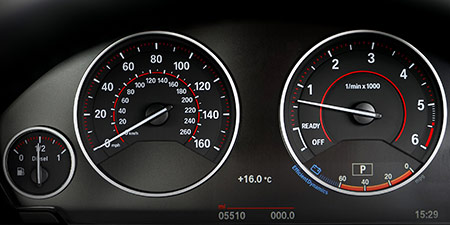# Speed of sound to Kilometers per hour (km/h)

Units of speed ﹣ Converter Speed of sound to Kilometers per hour

Here you can convert the Speed unit Speed of sound into the unit Kilometers per hour and vice versa you can convert Kilometers per hour into Speed of sound. By clicking the "Swap units" icon, you will always obtain the desired conversion in the calculation result. With the following calculator you can also calculate any other Speed unit.

## Info about "Speed of sound"

Definition: If an object travels at the speed of sound in air at 20 degrees Celsius for one second, it travels a distance of 343 meters per second. Actually, it is equivalent to 1.234,8 km/h.

The speed of sound is often illustrated by an example of a thunderstorm: if lightning strikes within 3 seconds of the thunder sound, then the thunderstorm is about 1 kilometer away. Another example, in a 100-meter sprint, the starting shot is fired directly next to the starting blocks. Imagine, if the start shot was fired at the finish line, it would take about 0.3 seconds of time to hear the shot in the start blocks.

## Info about "Kilometers per hour"

Kilometers per hour is used as a standard unit in transportation, therefore it is used in traffic or to measure the travel speed of vehicles. For example, a commercial aircraft flies at a speed of 1,000 km/h then it covers a distance of 1,000 kilometers per hour.

The unit is defined as follows: An object moving at 1 "km/h" per hour will cover one kilometer. "km/h" is approved to use in the international system of units (SI).

## Formula for the conversion of Speed of sound to Kilometers per hour (km/h) and vice versa

The calculation from Speed of sound to Kilometers per hour shall be made using the following conversion formula:

Conversion formula Speed of sound to Kilometers per hour

Determine the number of Kilometers per hour from Speed of sound

Speed of sound × 1234.8

## Formula for the conversion of Kilometers per hour (km/h) to Speed of sound

The calculation from Kilometers per hour to Speed of sound shall be made using the following conversion formula:

Conversion formula Kilometers per hour to Speed of sound

Determine the number of Speed of sound from Kilometers per hour

Kilometers per hour × 0.00080984774862326

## Overview table : How many Speed of sound are in a Kilometers per hour ?

Speed of sound ⇒ Kilometers per hour km/h
0.01   are  12.34800 km/h
0.02   are  24.69600 km/h
0.03   are  37.04400 km/h
0.04   are  49.39200 km/h
0.05   are  61.74000 km/h
0.06   are  74.08800 km/h
0.07   are  86.43600 km/h
0.08   are  98.78400 km/h
0.09   are  111.13200 km/h
0.10   are  123.48000 km/h
0.20   are  246.96000 km/h
0.30   are  370.44000 km/h
0.40   are  493.92000 km/h
0.50   are  617.40000 km/h
0.60   are  740.88000 km/h
0.70   are  864.36000 km/h
0.80   are  987.84000 km/h
0.90   are  1 111.32000 km/h
1   corresponds to  1 234.80000 km/h
2   are  2 469.60000 km/h
3   are  3 704.40000 km/h
4   are  4 939.20000 km/h
5   are  6 174 km/h
6   are  7 408.80000 km/h
7   are  8 643.60000 km/h
8   are  9 878.40000 km/h
9   are  11 113.20000 km/h
10   are  12 348 km/h
20   are  24 696 km/h
30   are  37 044 km/h
40   are  49 392 km/h
50   are  61 740 km/h
60   are  74 088 km/h
70   are  86 436 km/h
80   are  98 784 km/h
90   are  111 132 km/h
100   are  123 480 km/h
200   are  246 960 km/h
300   are  370 440 km/h
400   are  493 920 km/h
500   are  617 400 km/h
600   are  740 880 km/h
700   are  864 360 km/h
800   are  987 840 km/h
900   are  1 111 320 km/h
1 000   are  1 234 800 km/h

## Overview table : How many Kilometers per hour are in a Speed of sound ?

Kilometers per hour km/h ⇒ Speed of sound
0.02 km/h  are  0.00001
0.03 km/h  are  0.00002
0.04 km/h  are  0.00003
0.05 km/h  are  0.00004
0.06 km/h  are  0.00004
0.07 km/h  are  0.00005
0.08 km/h  are  0.00006
0.09 km/h  are  0.00007
0.10 km/h  are  0.00008
0.20 km/h  are  0.00016
0.30 km/h  are  0.00024
0.40 km/h  are  0.00032
0.50 km/h  are  0.00040
0.60 km/h  are  0.00048
0.70 km/h  are  0.00056
0.80 km/h  are  0.00064
0.90 km/h  are  0.00072
1 km/h  corresponds to  0.00081
2 km/h  are  0.00162
3 km/h  are  0.00243
4 km/h  are  0.00323
5 km/h  are  0.00404
6 km/h  are  0.00485
7 km/h  are  0.00566
8 km/h  are  0.00647
9 km/h  are  0.00728
10 km/h  are  0.00809
20 km/h  are  0.01619
30 km/h  are  0.02429
40 km/h  are  0.03239
50 km/h  are  0.04049
60 km/h  are  0.04859
70 km/h  are  0.05668
80 km/h  are  0.06478
90 km/h  are  0.07288
100 km/h  are  0.08098
200 km/h  are  0.16197
300 km/h  are  0.24295
400 km/h  are  0.32393
500 km/h  are  0.40492
600 km/h  are  0.48590
700 km/h  are  0.56689
800 km/h  are  0.64787
900 km/h  are  0.72886
1 000 km/h  are  0.80984